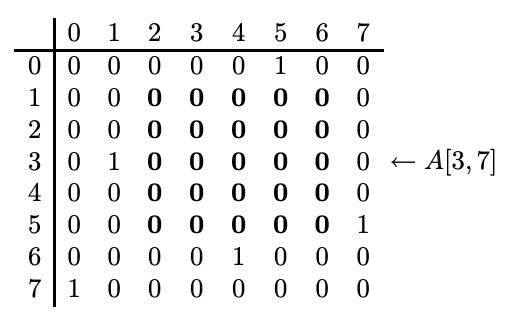시간 제한 메모리 제한 제출 정답 맞은 사람 정답 비율
2 초 512 MB 0 0 0 0.000%

## 문제

A group of scientists want to monitor a huge forest. They plan to airdrop small sensors to the forest. Due to many unpredictable conditions during airdropping, each sensor will land in a random location in the forest. After all sensors have landed, there will be square regions in the forest that do not contain any sensor. Let us call such a region a hole. It is desirable that all holes are small. This can be achieved by airdropping very large number of sensors. On the other hand, those sensors are expensive. Hence, the scientists want to conduct a computer simulation to determine how many sensors should be airdropped, so that the chances of having a large hole are small. To conduct this simulation, a subroutine is required that, given the locations of the sensors, outputs the size of the largest hole. This subroutine has to be very efficient (i.e. fast) since the simulation will be repeated many times with different parameters. You are tasked to write this efficient subroutine.

Consider an n by n array A of 0 and 1’s. We say that there is a hole in A at (x, y) with width d if the value of A[i, j] is 0, where

i = x,(x + 1),(x + 2), . . . ,(x + d − 1)
j = y,(y + 1),(y + 2), . . . ,(y + d − 1),

and (x + d − 1) < n,(y + d − 1) < n. That is, all values within the “square” at (x, y) with width d are 0’s. Note that the indices of the array start from 0.

Given the array A, we want to find the width of the largest hole.

## 입력

The input represents the input array A. The following is the input file for the array shown above.

The first line contains a positive integer n ≤ 1,024 which is the width of the array. The second line contains an integer k, which is the number of 1’s in the array. Next, it is followed by k more lines which specify the entries with value 1. Each line contains two integers x and y, which indicates that A[x, y] = 1.

Since the value of n may be as large as 1,024, your program has to run fast enough to handle arrays of that size.

## 출력

The output consists of an integer, which is the width of the largest hole. If there is no hole in the array, the output is 0. The following is the output of our example.

## 예제 입력 1

8
5
3 1
7 0
6 4
0 5
5 7


## 예제 출력 1

5


## 힌트

The following figure shows an array where the entry A[i, j] is at the i-th row and j-th column. The arrow points to the location of A[3, 7].

In this array, there is a hole of width 3 at (0,0), and it is not possible to have a hole of width 4 at (0,0). The largest hole has width 5 and it is at (1,2), which is highlighted in the figure.There are different fast methods that find the largest hole. Below are hints to two different methods.

Hint 1: Consider these 2 values:

• d1: the width of the largest possible hole at (1,1), and
• d2: the width of the largest possible hole at (2,2).

What is the relationship between d1 and d2? Suppose we know the value of d2, is there a fast method to compute d1?

Hint 2: Consider these 2 values:

• s1: the number of 1’s within the square at (0,0) with width 7, and
• s2: the number of 1’s within the square at (0,1) with width 7.

What is the relationship between s1 and s2? Suppose we know the value of s1, is there a fast method to compute s2?